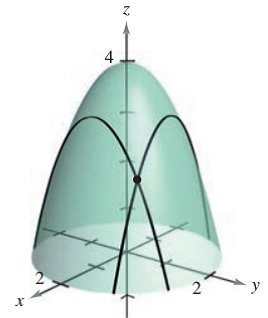Chapter 7.4, Problem 27E### Calculus: An Applied Approach (Min...

10th Edition
Ron Larson
ISBN: 9781305860919

#### Solutions

Chapter
Section### Calculus: An Applied Approach (Min...

10th Edition
Ron Larson
ISBN: 9781305860919
Textbook Problem
1 views

# Finding Slopes in the x- and y-Directions In Exercises 25-28, find the slopes of the surface at the given point in (a) the x-direction and (b) the y-direction. See Example 3. z = 4 − x 2 − y 2 ( 1 , 1 , 2 )(a)

To determine

To calculate: The slope of the surface z=4x2y2 in x direction at the point (1,1,2).

Explanation

Given information:

The provided function is z=4x2y2 and the point is (1,1,2).

Formula used:

Consider the function z=f(x,y) then for the value of zx consider y to be constant and differentiate with respect to x and the value of zy consider x to be constant and differentiate with respect to y.

Calculation:

Consider the provided function is,

z=4x2y2

Partially derivative of the function z=4x2y2 with respect to x.

zx=x(4x2y2)=x(4)x

(b)

To determine

To calculate: The slope of the surface z=4x2y2 in x direction at the point (1,1,2).

### Still sussing out bartleby?

Check out a sample textbook solution.

See a sample solution

#### The Solution to Your Study Problems

Bartleby provides explanations to thousands of textbook problems written by our experts, many with advanced degrees!

Get Started

#### Is there a number that is exactly 1 more than its cube?

Single Variable Calculus: Early Transcendentals, Volume I

#### In Exercises 63-68, use the graph of the function f to determine limxf(x) and limxf(x) 65.

Applied Calculus for the Managerial, Life, and Social Sciences: A Brief Approach

#### In problems 49-54, rationalize the denominator and then simpify. 52.

Mathematical Applications for the Management, Life, and Social Sciences

#### ∫x−4dx = −4x−5 + C

Study Guide for Stewart's Single Variable Calculus: Early Transcendentals, 8th

#### Explain how inter-rater reliability is established.

Research Methods for the Behavioral Sciences (MindTap Course List)